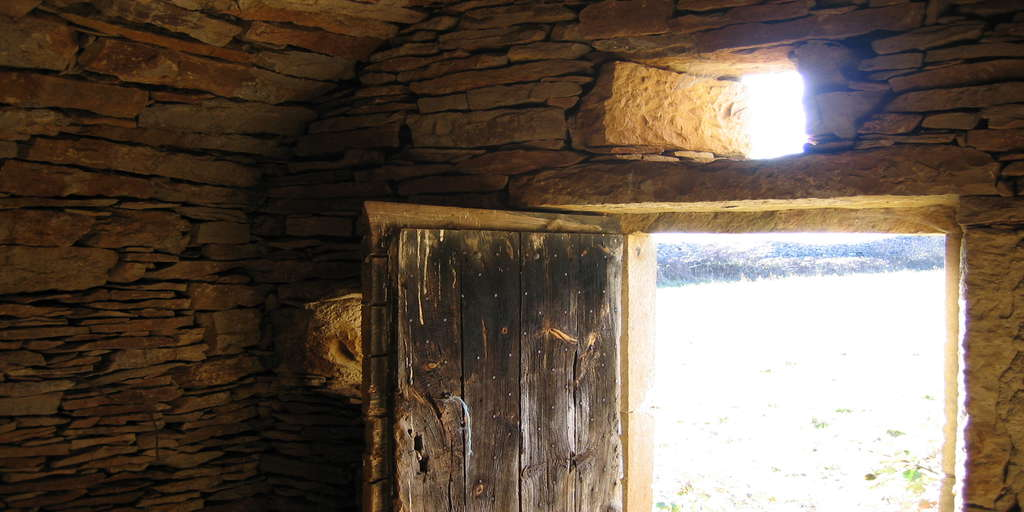# Clustering Example: 4 Steps You Should Know#### Clustering Example: 4 Steps You Should Know

This article describes k-means clustering example and provide a step-by-step guide summarizing the different steps to follow for conducting a cluster analysis on a real data set using R software.

We’ll use mainly two R packages:

• cluster: for cluster analyses and
• factoextra: for the visualization of the analysis results.

Install these packages, as follow:

install.packages(c("cluster", "factoextra"))

A rigorous cluster analysis can be conducted in 3 steps mentioned below:

Here, we provide quick R scripts to perform all these steps.

Contents:

#### Related Book

Practical Guide to Cluster Analysis in R

## Data preparation

We’ll use the demo data set USArrests. We start by standardizing the data using the scale() function:

# Load the data set
data(USArrests)
# Standardize
df <- scale(USArrests)

## Assessing the clusterability

The function get_clust_tendency() [factoextra package] can be used. It computes the Hopkins statistic and provides a visual approach.

library("factoextra")
res <- get_clust_tendency(df, 40, graph = TRUE)
# Hopskin statistic
res$hopkins_stat ##  0.656 # Visualize the dissimilarity matrix print(res$plot)The value of the Hopkins statistic is significantly < 0.5, indicating that the data is highly clusterable. Additionally, It can be seen that the ordered dissimilarity image contains patterns (i.e., clusters).

## Estimate the number of clusters in the data

As k-means clustering requires to specify the number of clusters to generate, we’ll use the function clusGap() [cluster package] to compute gap statistics for estimating the optimal number of clusters . The function fviz_gap_stat() [factoextra] is used to visualize the gap statistic plot.

library("cluster")
set.seed(123)
# Compute the gap statistic
gap_stat <- clusGap(df, FUN = kmeans, nstart = 25,
K.max = 10, B = 100)
# Plot the result
library(factoextra)
fviz_gap_stat(gap_stat)The gap statistic suggests a 4 cluster solutions.

It’s also possible to use the function NbClust() [in NbClust] package.

## Compute k-means clustering

K-means clustering with k = 4:

# Compute k-means
set.seed(123)
km.res <- kmeans(df, 4, nstart = 25)
head(km.res$cluster, 20) ## Alabama Alaska Arizona Arkansas California Colorado ## 4 3 3 4 3 3 ## Connecticut Delaware Florida Georgia Hawaii Idaho ## 2 2 3 4 2 1 ## Illinois Indiana Iowa Kansas Kentucky Louisiana ## 3 2 1 2 1 4 ## Maine Maryland ## 1 3 # Visualize clusters using factoextra fviz_cluster(km.res, USArrests)## Cluster validation statistics: Inspect cluster silhouette plot Recall that the silhouette measures ($S_i$) how similar an object $i$ is to the the other objects in its own cluster versus those in the neighbor cluster. $S_i$ values range from 1 to - 1: • A value of $S_i$ close to 1 indicates that the object is well clustered. In the other words, the object $i$ is similar to the other objects in its group. • A value of $S_i$ close to -1 indicates that the object is poorly clustered, and that assignment to some other cluster would probably improve the overall results. sil <- silhouette(km.res$cluster, dist(df))
rownames(sil) <- rownames(USArrests)
head(sil[, 1:3])
##            cluster neighbor sil_width
## Alabama          4        3    0.4858
## Alaska           3        4    0.0583
## Arizona          3        2    0.4155
## Arkansas         4        2    0.1187
## California       3        2    0.4356
## Colorado         3        2    0.3265
fviz_silhouette(sil)
##   cluster size ave.sil.width
## 1       1   13          0.37
## 2       2   16          0.34
## 3       3   13          0.27
## 4       4    8          0.39It can be seen that there are some samples which have negative silhouette values. Some natural questions are :

Which samples are these? To what cluster are they closer?

This can be determined from the output of the function silhouette() as follow:

neg_sil_index <- which(sil[, "sil_width"] < 0)
sil[neg_sil_index, , drop = FALSE]
##          cluster neighbor sil_width
## Missouri       3        2   -0.0732

## eclust(): Enhanced clustering analysis

The function eclust()[factoextra package] provides several advantages compared to the standard packages used for clustering analysis:

• It simplifies the workflow of clustering analysis
• It can be used to compute hierarchical clustering and partitioning clustering in a single line function call
• The function eclust() computes automatically the gap statistic for estimating the right number of clusters.
• It automatically provides silhouette information
• It draws beautiful graphs using ggplot2

### K-means clustering using eclust()

# Compute k-means
res.km <- eclust(df, "kmeans", nstart = 25)# Gap statistic plot
fviz_gap_stat(res.km\$gap_stat)# Silhouette plot
fviz_silhouette(res.km)### Hierachical clustering using eclust()

 # Enhanced hierarchical clustering
res.hc <- eclust(df, "hclust") # compute hclust
## Clustering k = 1,2,..., K.max (= 10): .. done
## Bootstrapping, b = 1,2,..., B (= 100)  [one "." per sample]:
## .................................................. 50
## .................................................. 100
fviz_dend(res.hc, rect = TRUE) # dendrogamThe R code below generates the silhouette plot and the scatter plot for hierarchical clustering.

fviz_silhouette(res.hc) # silhouette plot
fviz_cluster(res.hc) # scatter plot

### Comments ( 2 )

•Gary Napier

Hi,

Great website, excellent content. But why do you have a huge banner at the top that comes almost a third of the way down the page when you scroll down? Particularly as this banner has so much wasted white space in it. Please change it, it’s driving me mad!

Thanks

•Thank you Gary for your suggestion. I’ll work in improving the appearance of the website as soon as possible.